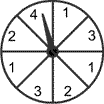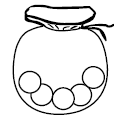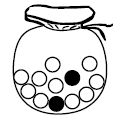Skill 26F
Probability: Likely, Unlikely, Certain, Impossible1. What number is the pointer most likely to land on?
 1 2 3 4
2. What number is the pointer least likely to land on?
 1 2 3 43. What is the probability of pulling a black marble?
 likely unlikely certain impossible
4. What's the probability of pulling a white marble?
 likely unlikely certain impossible5. What's the probability of pulling a white marble?
 likely unlikely certain impossible
6. What's the probability of pulling a black marble?
 likely unlikely certain impossible